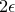# B.1 The typesetting of text

Pyxplot renders all text labels automatically in the latex typesetting environment. This brings many advantages: it produces neater labels than the default typesetting engine used by gnuplot, makes it straightforward to label graphs with mathematical expressions, and moreover makes it straightforward when importing graphs into latex documents to match the fonts used in figures with those used in the main text of the document. It does, however, also necessarily introduce some incompatibility with gnuplot. Some strings which are valid in gnuplot are not valid in Pyxplot (see Section 3.6 for more details). For example,set xlabel ’x2’

is a valid label in gnuplot, but is not valid input for latex and therefore fails in Pyxplot. In Pyxplot, it needs to be written in latex mathmode as:set xlabel ’$x2$’

A useful introduction to latex’s syntax can be found in Tobias Oetiker’s excellent free tutorial, The Not So Short Guide to latex, which is available for free download from:

http://www.ctan.org/tex-archive/info/lshort/english/lshort.pdf

Two built-in functions provide some assistance in generating latex labels. The texify() takes as its argument a string containing a mathematical expression, and returns a latex representation of it. The texifyText() takes as its argument a text string, and returns a latex representation of it, with any necessary escape characters added. For example:

pyxplot> print texify("sqrt(x**2+1)")
$displaystylesqrt{x,*kern-1.5pt *,,2+1}mathrm{}$
pyxplot> a=50
pyxplot> print texifyText("A %d%% increase"%a)
A 50% increase
pyxplot> set ylabel texify("cos(x**2)") Two built-in functions provide some assistance in generating latex labels. The texify() takes as its argument a string containing a mathematical expression, and returns a latex representation of it. The texifyText() takes as its argument a text string, and returns a latex representation of it, with any necessary escape characters added. For example:

pyxplot> print texify("sqrt(x**2+1)")
$displaystylesqrt{x,*kern-1.5pt *,,2+1}mathrm{}$
pyxplot> a=50
pyxplot> print texifyText("A %d%% increase"%a)
A 50% increase
pyxplot> set ylabel texify("cos(x**2)")